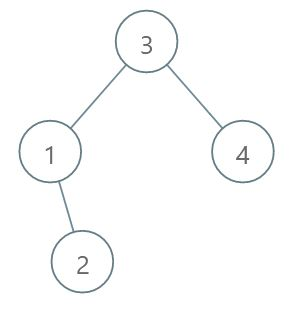# Second Largest Node in Binary Search Tree

## Problem

You are given the root to a binary search tree. Find the second largest node in the tree and return it. For example for the given binary search tree, return 3.## Solution

It may be noted that in the following solutions, NULL is returned if there are less than 2 nodes in the BST.

### Recursive Solution

Inorder traversal traverses the BST in a sorted way. Thus, if we perform a reverse inorder traversal, we will get the largest node first and then second largest node and so on.

In the following solution k is set to 2, since we want to find the second largest node. In the reverse inorder traversal function, k is decremented every time we are at the current node (revere inorder traversal is performed by visiting right node first, then current node and then the left node). As soon as k is 0, the value of the current node is returned.

int secondLargestElement = NULL;
void reverseInorder(TreeNode* root, int &k) {
if (root == nullptr) return;
reverseInorder(root -> right, k);
--k;
if (k == 0) {
secondLargestElement = root -> val;
return;
}
reverseInorder(root -> left, k);
}

int secondLargestRecursive(TreeNode* root) {
int k = 2;
reverseInorder(root, k);
return secondLargestElement;
}
• Time Complexity: $O(h)$, where $h$ is the height of the BST which can go as maximum as the number of nodes for a skewed BST.
• Space Complexity: $O(h)$ (where $h$ is the height of the BST) for the implicit stack required for recursion.

### Iterative solution

For iterative solution we have to consider few cases:

• Right subtree is empty: The answer will be inorder predecessor to the root.
• Right-most node is not a leaf node: The answer will be the left child of the right most node.
• Right-most node is a leaf node: The answer will be the parent to the right-most node.
int secondLargestIterative(TreeNode* root) {
TreeNode* prevNode;
// empty BST
if (!root) return NULL;
// less than 2 nodes in the BST
if (!root -> right and !root -> left) return NULL;
// right subtree is empty
if (!root -> right) {
root = root -> left;
while(root -> right) {
root = root -> right;
}
return root -> val;
}
// traverse to the right most node
while(root -> right) {
prevNode = root;
root = root -> right;
}
// empty right most node
if (!root -> right and !root -> left) {
return prevNode -> val;
}
return root -> left -> val;
}

Time Complexity: $O(h)$, where $h$ is the height of the BST which can go as maximum as the number of nodes for a skewed BST.

Space Complexity: $O(1)$.